• 本程序是窗函数法设计FIR数字滤波器Matlab程序——矩形窗
• matlab代码，直接运行出结果。FIR带通滤波器，并对数字信号进行带通滤波。建立含有3种频率和白噪声的信号。然后利用窗函数法设计
• 本程序是窗函数法设计FIR数字滤波器Matlab程序——汉宁窗
• 程序是利用matlab编写，结合barrtlet滤波器，实现FIR数字滤波的一个程序FIR滤波
• 本程序是窗函数法设计FIR数字滤波器Matlab程序——布莱克曼窗
• 程序包中分别使用最小二乘法，拉格朗日插值法，优化加权最小二乘法来实现分数延迟FIR滤波器Matlab编程
• matlab fir滤波器设计 Matlab结合窗函数法设计数字带通FIR滤波器课程设计任务书学生姓名： 专业班级： 通信工程 指导教师： 工作单位： 信息工程学院 题 目:利用Matlab仿真软件系统结合窗函数法设计一个数字带通FIR...

matlab fir滤波器设计 Matlab结合窗函数法设计数字带通FIR滤波器

课程设计任务书学生姓名： 专业班级： 通信工程 指导教师： 工作单位： 信息工程学院 题 目:利用Matlab仿真软件系统结合窗函数法设计一个数字带通FIR滤波器 初始条件： 1.《数字信号处理》基本理论知识2.《信号与系统》基本理论知识3.MATL…

电子系统设计实验报告 姓 名 杨熙丞 指导教师 贾立新 专业班级 自动化1201 学 院 信息工程学院 一．设计题目设计一4阶带通滤波器，通带范围为300Hz~10kHz，通带增益A0=1.二．原理图设计此次设计的带通滤波器，上限频率fH?3400…

《数字信号处理课程设计报告》 题 目： IIR数字带通滤波器设计学 院：专 业：班 级：姓 名：指导教师： 2012年 6月24日 目 录 1数字滤波器设计原理…………………………………………………31.1数字滤波器简介 ………………………………………

课程设计任务书

学生姓名： 专业班级： 通信工程 指导教师： 工作单位： 信息工程学院 题 目:利用Matlab仿真软件系统结合窗函数法设计一个数字带通FIR滤波器 初始条件： 1.《数字信号处理》基本理论知识

2.《信号与系统》基本理论知识

3.MATLAB编程基础知识 4.装有MATLAB的PC机

要求完成的主要任务:

利用MATLAB仿真软件系统结合窗函数法设计一个数字带通FIR滤波器

时间安排：

第20周理论设计、实验室安装调试，地点： 鉴主15楼通信实验室一

指导教师签名： 年 月 日

系主任(或责任教师)签名： 年 月 日

目录

目录 ......................................................................... 中文摘要 .................................................................... 1 Abstract .................................................................... 2 1 MATLAB的概况 ............................................................. 3 2 MATLAB结合窗函数设计法原理 ............................................... 4 3 各类窗函数简介 ............................................................ 7

3.1 基本窗函数 ......................................................................................................................... 7

3.1.1 矩形窗函数 ............................................................................................................... 7 3.1.2三角窗 ........................................................................................................................ 7 3.2 广义余弦窗 ......................................................................................................................... 8

3.2.1汉宁窗(升余弦窗) ................................................................................................... 9

4 方案设计与论证 ........................................................... 10

4.1 程序设计法

展开全文• 本程序是窗函数法设计FIR数字滤波器Matlab程序——海明窗
• 1. 采用fir1函数设计，fir1函数可以设计低通、带通、高通、带阻等多种类型的具有严格线性相位特性的FIR滤波器。语法形式：b = fir1(n, wn)b = fir1(n, wn, ‘ftype’)b = fir1(n, wn, ‘ftype’, window)b = fir1(n,...

1.      采用fir1函数设计，fir1函数可以设计低通、带通、高通、带阻等多种类型的具有严格线性相位特性的FIR滤波器。语法形式：

b = fir1(n, wn)

b = fir1(n, wn, ‘ftype’)

b = fir1(n, wn, ‘ftype’, window)

b = fir1(n, wn, ‘ftype’, window, ‘noscale’)

参数的意义及作用：

b：返回的FIR滤波器单位脉冲响应，脉冲响应为偶对称，长度为n+1；

n：滤波器的介数；

wn：滤波器的截止频率，取值范围为0

window：指定使用的窗函数，默认为海明窗；

noscale：指定是否归一化滤波器的幅度。

示例：

N=41;      %滤波器长度

fs=2000;   %采样频率

%各种滤波器的特征频率

fc_lpf=200;

fc_hpf=200;

fp_bandpass=[200 400];

fc_stop=[200 400];

%以采样频率的一半，对频率进行归一化处理

wn_lpf=fc_lpf*2/fs;

wn_hpf=fc_hpf*2/fs;

wn_bandpass=fp_bandpass*2/fs;

wn_stop=fc_stop*2/fs;

%采用fir1函数设计FIR滤波器

b_lpf=fir1(N-1,wn_lpf);

b_hpf=fir1(N-1,wn_hpf,‘high‘);

b_bandpass=fir1(N-1,wn_bandpass,‘bandpass‘);

b_stop=fir1(N-1,wn_stop,‘stop‘);

%求滤波器的幅频响应

m_lpf=20*log(abs(fft(b_lpf)))/log(10);

m_hpf=20*log(abs(fft(b_hpf)))/log(10);

m_bandpass=20*log(abs(fft(b_bandpass)))/log(10);

m_stop=20*log(abs(fft(b_stop)))/log(10);

%设置幅频响应的横坐标单位为Hz

x_f=0:(fs/length(m_lpf)):fs/2;

%绘制单位脉冲响应

subplot(421);stem(b_lpf);xlabel(‘n‘);ylabel(‘h(n)‘);

subplot(423);stem(b_hpf);xlabel(‘n‘);ylabel(‘h(n)‘);

subplot(425);stem(b_bandpass);xlabel(‘n‘);ylabel(‘h(n)‘);

subplot(427);stem(b_stop);xlabel(‘n‘);ylabel(‘h(n)‘);

%绘制幅频响应曲线

subplot(422);plot(x_f,m_lpf(1:length(x_f)));xlabel(‘频率(Hz)‘,‘fontsize‘,8);ylabel(‘幅度(dB)‘,‘fontsize‘,8);

subplot(424);plot(x_f,m_hpf(1:length(x_f)));xlabel(‘频率(Hz)‘,‘fontsize‘,8);ylabel(‘幅度(dB)‘,‘fontsize‘,8);

subplot(426);plot(x_f,m_bandpass(1:length(x_f)));xlabel(‘频率(Hz)‘,‘fontsize‘,8);ylabel(‘幅度(dB)‘,‘fontsize‘,8);

subplot(428);plot(x_f,m_stop(1:length(x_f)));xlabel(‘频率(Hz)‘,‘fontsize‘,8);ylabel(‘幅度(dB)‘,‘fontsize‘,8);2.      采用fir2函数设计，函数算法是：首先根据要求的幅频响应向量形式进行插值，然后进行傅里叶变换得到理想滤波器的单位脉冲响应，最后利用窗函数对理想滤波器的单位脉冲响应激进型截断处理，由此得到FIR滤波器系数。fir2函数的语法形式：

b = fir2(n, f, m)

b = fir2(n, f, m, window)

b = fir2(n, f, m, npt)

b = fir2(n, f, m, npt, window)

b = fir2(n, f, m, npt, lap)

b = fir2(n, f, m, npt, lap, window)

各项参数的意义及作用：

n及b：滤波器的介数，返回值b为滤波器系数，长度为n+1。注意：当设计的滤波器在归一化频率为1处的幅度值不为0时，n不能为奇数；

f及m：f取值在0~1之间，对应为滤波器的归一化频率；m是长度与f相同的向量，用于设置对应频段范围内的理想幅值；

window：用于指定窗函数的种类，默认是海明窗；

npt：正整数，用于指定对幅度响应进行插值时的插值点个数，默认是512；

lap：正整数，用于指定对幅度响应进行插值时，对于不连续点转变成连续时的点数，默认25

示例：

N=120;                  %滤波器阶数

fc=[0 0.125 0.125 0.25 0.25 0.5 0.5 1];   %截止频率

mag=[1 1 0.5 0.5 0.25 0.25 0.125 0.125 ]; %理想滤波器幅度

b=fir2(N,fc,mag);      %设计海明窗滤波器

freqz(b);              %绘制频率响应曲线3.      采用kaiserord函数设计，凯塞窗具有可调参数选项，可根据相关算法，先选择过渡带、容限参数，并根据这些参数计算出凯塞窗的值，以及滤波器阶数，语法形式：

[n, wn, beta, filtype] = kaiserord(f, a, dev, fs)

各项参数的意义和作用：

f及fs：如果f是一个向量，则其中的元素是待设计的过渡带的起始点和结束点；如果没有fs参数，f中元素的取值范围是0~1，即相对于采样频率一半的归一化频率；如果有fs参数，则fs为信号采样频率，f中元素即为实际的截止频率；

a：是一个向量，用于指定过渡带频率段的理想幅度值；

dev：是一个向量，用于指定通带或阻带内的容许误差；

n：设计的最小阶数；

wn：向量，计算得到的滤波器截止频率点；

beta：计算得到的值；

ftype：根据设计要求得到的滤波器类型参数

4.      采用firpm函数设计，采用最大误差最小准则进行滤波器设计，语法形式：

b = firpm(n, f, a)

b = firpm(n, f, a,w)

b = firpm(n, f, a,’ftype’)

b = firpm(n, f, a, w, ‘ftype’)

[b, delta] = firpm(…)

各项参数的意义及作用：

n及b：滤波器阶数；

f及a：两个长度相同向量，f取值0~1之间，对应滤波器的归一化频率，a用于设置对应频段范围内的理想幅值；

w：长度为f的一半，实现对应频段幅度值的权值；

ftype：指定滤波器结构类型，如果没有设置该参数，表示设计偶对称脉冲响应滤波器；如果设置为hilbert，则表示设计奇对称结构的滤波器，具有相移特性；

delta：返回滤波器最大容限值

示例：

利用凯塞窗函数设计低通FIR滤波器，过渡带为1000~1500Hz，采样频率为8000Hz，通带容限最大为0.01，阻带容限最大为0.05.利用海明窗及firpm函数设计相同的低通滤波器，截止频率为1500Hz，滤波器阶数为凯塞窗函数求取的值。

fs=8000;         %采样频率

fc=[1000 1500];  %过渡带

mag=[1 0];       %窗函数的理想滤波器幅度

dev=[0.01 0.05]; %纹波

[n,wn,beta,ftype]=kaiserord(fc,mag,dev,fs);  %获取凯塞窗参数

fpm=[0 fc(1)*2/fs fc(2)*2/fs 1];  %firpm函数的频段向量

magpm=[1 1 0 0];                  %firpm函数的幅值向量

%设计凯塞窗及海明窗滤波器

h_kaiser=fir1(n,wn,ftype,kaiser(n+1,beta));

h_hamm=fir1(n,fc(2)*2/fs);

%设计最优滤波器

h_pm=firpm(n,fpm,magpm);

%求滤波器的幅频响应

m_kaiser=20*log(abs(fft(h_kaiser,1024)))/log(10);

m_hamm=20*log(abs(fft(h_hamm,1024)))/log(10);

m_pm=20*log(abs(fft(h_pm,1024)))/log(10);

%设置幅频响应的横坐标单位为Hz

x_f=[0:(fs/length(m_kaiser)):fs/2];

%只显示正频率部分的幅频响应

m1=m_kaiser(1:length(x_f));

m2=m_hamm(1:length(x_f));

m3=m_pm(1:length(x_f));

%绘制幅频响应曲线

plot(x_f,m1,‘-‘,x_f,m2,‘-.‘,x_f,m3,‘--‘);

xlabel(‘频率(Hz)‘);ylabel(‘幅度(dB)‘);

legend(‘凯塞窗‘,‘海明窗‘,‘最优滤波器‘);

grid;展开全文• fir滤波器matlab程序，包括低通、高通、带阻、带通，注释非常详尽，大家可以对里面的相关参数进行修改，比如截止频率，窗口类型等，而且对滤波前后波形进行了频谱分析，以验证滤波的效果，非常直观，很有利于初学者...
• 用MATLAB设计FIR滤波器

千次阅读 多人点赞 2021-01-28 13:10:07
用MATLAB设计FIR滤波器滤波器滤波器定义滤波器种类滤波器设计滤波器设计要求Matlab程序设计滤波器利用Matlab工具箱设计滤波器总结 滤波器 滤波器定义 “滤波器(filter),是一种用来消除干扰杂讯的器件,将输入或输出...

滤波器

滤波器定义

“滤波器(filter),是一种用来消除干扰杂讯的器件,将输入或输出经过过滤而得到纯净的直流电。对特定频率的频点或该频点以外的频率进行有效滤除的电路,就是滤波器,其功能就是得到一个特定频率或消除一个特定频率。”

滤波器种类

滤波器按照频率来分类，可分为高通、低通、带通、带阻以及全通滤波器，根据所需选择合适滤波器。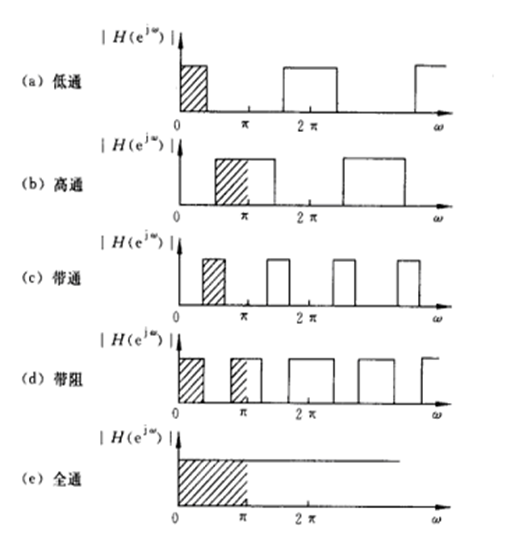滤波器设计

滤波器的设计方法可分为两大类，一类是IIR，另一类是FIR。对于FIR的设计，一般可以采用等波纹以及窗的方法。

滤波器设计要求

采样频率50khz，带通滤波器，通带15KHz，阻带20KHz，阻带衰减50dB，用凯撒窗设计带通滤波器。

Matlab程序设计滤波器

首先在Matlab中设置所需参数：

fs = 50000;
T = 1/fs;
L = 4000;
t = (0:L-1)*T;

然后从r32文件中读取信号数据：

filename=['文件路径'];
fid=fopen(filename,'r');
Na=4000;
data=dat(1,:); %data即为所导入信号

绘制信号时域图：

plot(t,data)

得到：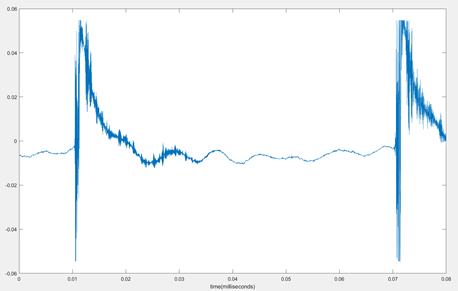再对其进行FFT：

NFFT = 2^nextpow2(L);
Y = fft(data,NFFT)/L;
f=fs/2*linspace(0,1,NFFT/2+1);
figure
plot(f,2*abs(Y(1:NFFT/2+1)))
title('Single-sided Amplitude Spectrum of y(t)')
xlabel('Frequency(Hz)')
ylabel('|Y(f)|')

得到：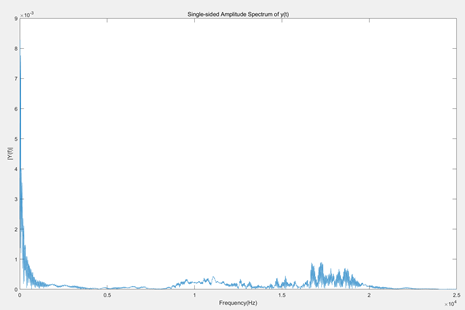设计滤波器：

fs = 50000;
f = [13000 15000 20000 22000];
dev = [0.01 0.02 0.01];
a = [0 1 0];
[n,wn,beta,ftype] = kaiserord(f,a,dev,fs);
b = fir1(n,wn,'bandpass');
freqz(b)

得到滤波器的幅值相位图：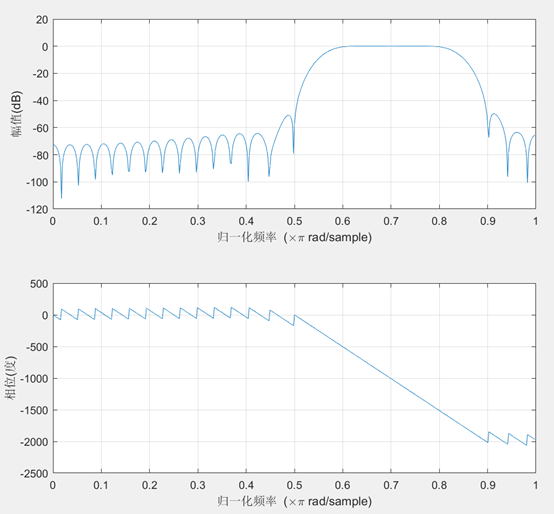所设计滤波器的分子系数存于b中，使所给信号通过所设计的滤波器，所用程序如下：

d=filter(b,1,data);
plot(t,d)

得到滤波后的信号时域图：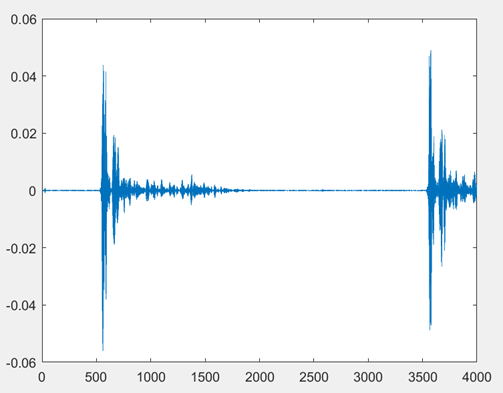对其进行FFT：

Y _af= fft(d,NFFT)/L;
f_af=fs/2*linspace(0,1,NFFT/2+1);
figure
plot(f_af,2*abs(Y_af(1:NFFT/2+1)))
xlabel('Frequency(Hz)')

得到：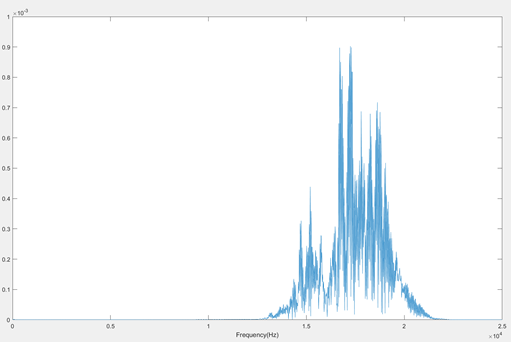利用Matlab工具箱设计滤波器

另外，还有一种更加快捷的设计方法，即使用Matlab自带的工具箱filterDesigner来设计滤波器：
在Matlab的命令行窗口中输入filterDesigner，得到如下窗口：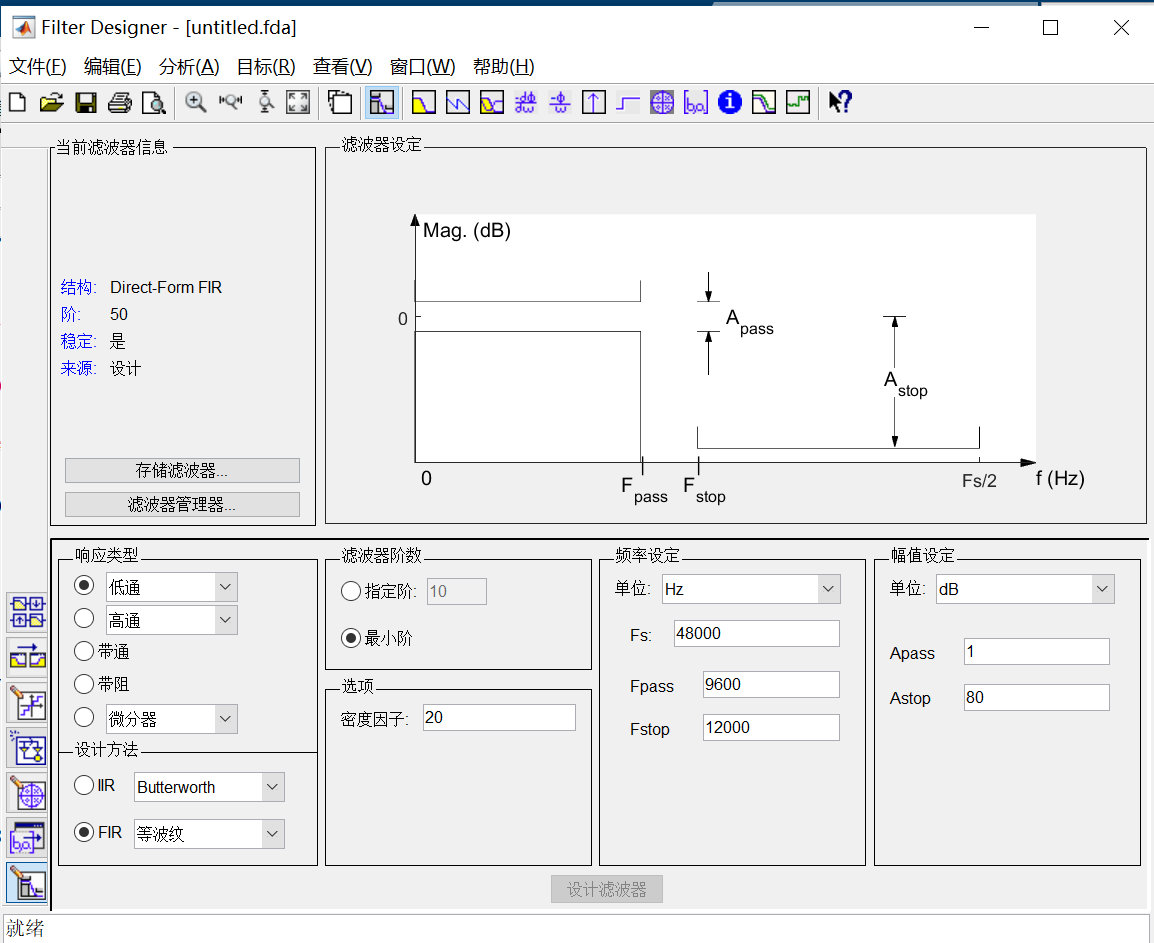通过选择设置，可以得到滤波器的系数，以本题为例：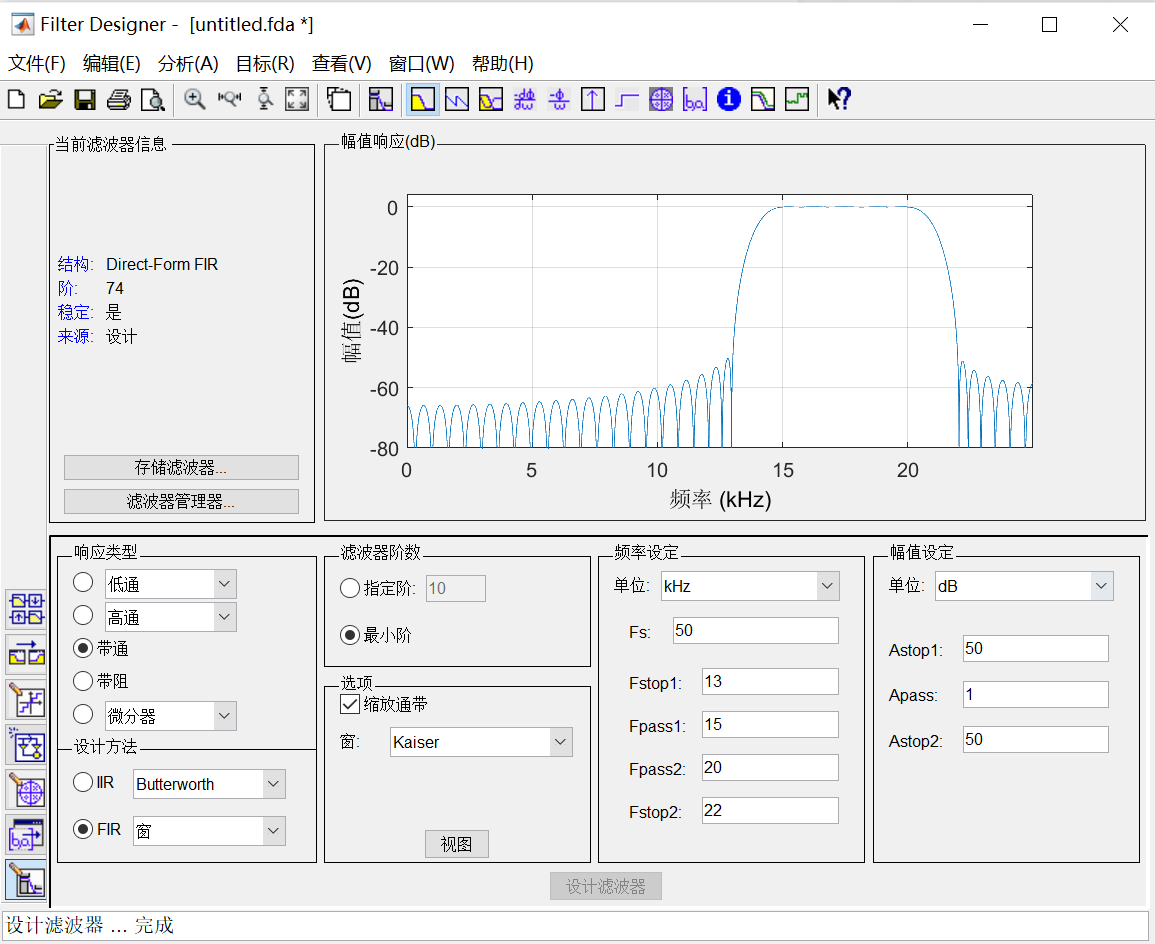其系数如下：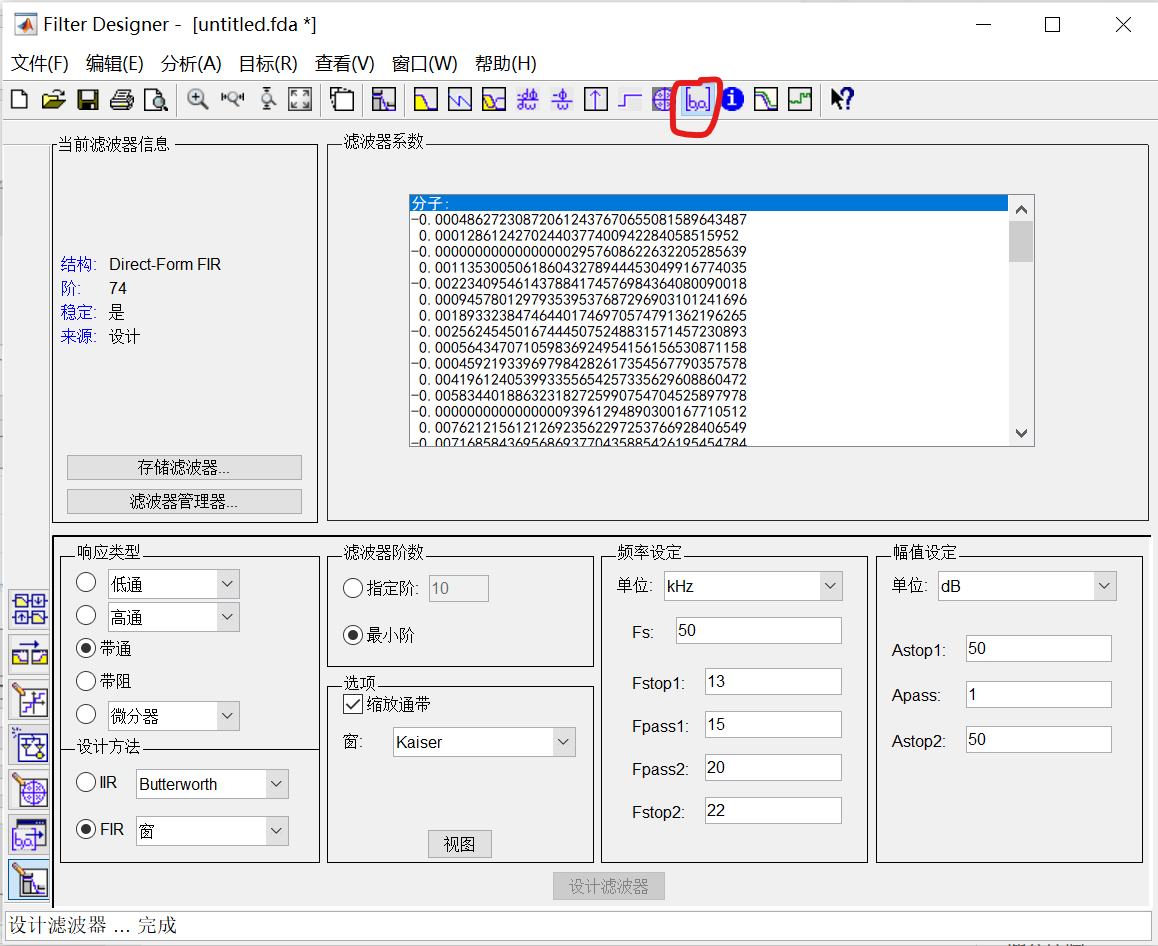可对其到处头文件，进行数据处理。

总结

本人对于数字信号处理这门课的学习比较冲忙，对于很多知识点都是比较模糊，希望在后续所需时能够进一步的加深理解。
在FIR滤波器设计的过程中，遇到一个比较困惑的点是，根据其他的案例，滤波器系数是包含分子系数以及分母系数，但本例产生的滤波器仅含分母系数，比较困惑，请大佬们指教！

展开全文matlab 数字信号处理
• 基于matlab的FIR数字滤波器设计学生姓名学 号所 在 系专业名称班 ...对于FIR数字滤波器的设计：本文分别用了窗函数设计法、最优等波动设计法、约束最小二乘法设计法、任意响应滤波器设计方法设计FIR滤波器。其中窗函...

基于matlab的FIR数字滤波器设计

学生姓名

学    号

所 在 系

专业名称

班    级

指导教师

摘 要

数字滤波器可以用软件或设计专用的数字处理硬件两种方式来实现，本文主要研究在MATLAB环境下，FIR数字滤波器的几种典型设计方法，并且分别对其进行仿真实现。

对于FIR数字滤波器的设计：本文分别用了窗函数设计法、最优等波动设计法、约束最小二乘法设计法、任意响应滤波器设计方法设计FIR滤波器。其中窗函数设计法重点在于选择某种恰当的窗函数和一种合适的理想滤波器，设计方法比较简便；最优等波动法解决了窗口法设计技术精度的困难，并使误差均匀分布；约束最小二乘法设计法使用了平方误差最小化的技术；而任意响应滤波器设计中可以设计任意复杂响应的FIR滤波器。各种设计方法都有利有弊，在实际设计中，可以通过设计要求来选择合适的设计FIR滤波器方法。

关键词: 数字滤波器；模拟滤波器；脉冲响应；双线性变换；幅频特性

Abstract

The digital filter may use the software or the design special-purpose digital processing hardware two ways realize, This article main research under MATLAB environment, FIR digital wave filter several model design method, And separately carries on the simulation experiment to it.

Design of FIR digital wave filter:Separately with window law, most excellent to fluctuate law of designing, restrain least square method from design law, wanton to respond design method of the wave filter design FIR wave filter.Among them window law lies in with emphasis chooses some kind of appropriate window law and one kind of appropriate ideal filter, The design method quite is simple; The most excellent fluctuate law has solved the window law design technology precision difficulty, And causes the erroneous uniform distribution; The restraint least squares method design law has used the square erroneous minimum technology; and wanton can design wanton complicated FIR wave filter that responded while responding the wave filter design. Each kind of design method all advantages are accompanied by disadvantages, In actual design,May choose the appropriate design FIR filter method through the design request.

Key words：Digital filter；Analog filter； The pulse is responded；

Pair is varied linearly；A characteristic frequently

目 录

摘 要 II

Abstract III

目 录 IV

第1章 概述 1

1.1 课题研究的目的和意义 1

1.2 国内外发展现状 1

1.3 本文的研究工作 2

第2章 MATLAB简介 3

2.1 MATLAB系统组成 3

2.2 MATLAB仿真简介 5

2.3 MATLAB 的M文件 6

第3章 数字滤波器 8

3.1 数字滤波器的分类 8

3.2 数字滤波器的设计简介 10

3.3 FIR滤波器特点 11

第4章FIR滤波器的设计 14

4.1 窗函数法设计FIR滤波器 14

4.2 最优等波动法设计FIR滤波器 23

4.3 约束最小二乘法设计FIR滤波器 26

4.4 任意响应法设计FIR滤波器 28

4.5 FIR滤波器设计实例 29

结 论 32

参考文献 33

致 谢 34

附 录 35

展开全文• matlab的FIR滤波器设计

万次阅读 多人点赞 2018-10-29 16:37:33
1.matlab设计fir滤波器的方法 2.fir1函数设计滤波器 clear all; close all; clc; % 滤波器长度 N=41; %采样频率 fs=2000; %各种滤波器的特征频率 fc_lpf=200; fc_hpf=200; fp_bandpass=[200 400]; fc_stop=[200 400]...matlab
• 窗函数设计FIR滤波，用C语言实现，在C环境下运行
• MATLAB设计FIR滤波器方法程序分享-FIR滤波器设计.doc 里面有低通，高通，带通滤波器的详细设计方法以及程序设计示例，希望对大家有帮助！matlab
• FIR滤波器的MATLAB与FPGA的设计实现

千次阅读 多人点赞 2019-10-10 16:13:07
FIR滤波器MATLAB与FPGA的设计实现 引言 数字滤波器是数字信号系统里面最常用、最重要、最基本的元件之一。有限脉冲响应(Finite Impulse Response,FIR)滤波器可以设计成任意幅频特性,同时保证精确，严格的线性...数字IC设计 FPGA MATLAB
• [Matlab]FIR滤波器设计：(基本窗函数FIR滤波器设计) ​ IIR滤波器主要设计方法先设计一个模拟低通滤波器，然后把它转化为形式上的数字滤波器。但对于FIR滤波器来说，设计方法的关键要求之一就是保证线性相位条件。而...FIR滤波器设计 数字信号处理
• 摘 要 介绍了利用 MATLAB 信号处理工具箱进行 FIR 滤波器设计的三种方法程序设计法 FDATool 设计法和 SPTool 设计法给出了详细的设计步骤并将设计的滤波器应用到一个 混和正弦波信号以验证滤波器的性能 关键词 ...
• matlab设计FIR 带通滤波器，实例讲解，程序代码，图像均包含。
• FDATOOL) ,根据指定的滤波器性能快速设计一个FIR ,再把滤波器系数以头文件形式导入CCS 中,头文件中MATLAB实现FIR 数字滤波器滤波器阶数和系数数组,在MATLAB中调试、运行程序并显示、分析处理后的数据。使用该方法,...
• 基于Matlab的FIR滤波器设计与实现

万次阅读 多人点赞 2017-10-21 18:31:22
前面一篇文章介绍了通过FDATool工具箱实现滤波器的设计，见“基于Matlab中FDATool工具箱的滤波器设计及相关文件的生成”，这里通过几个例子说明采用Matlab语言设计FIR滤波器的过程。 二、实验平台 　Matlab...
• 今天和大侠简单聊一聊基于matlab FPGA verilog的FIR滤波器设计，话不多说，上货。 本次设计实现8阶滤波器，9个系数，由于系数的对称性，h(0)=h(8)，h1(1)=h(7)，h(2)=h(6)，h(3)=h(5)，h(4)为中间单独一个系数。...FIR滤波器设计 fpga matlab
• 《基于matlabFIR滤波器设计(高通...1、基于matlabFIR滤波器设计(高通，频率取样法)一、参考程序M=32;%所需频率采样点个数Wp=0.6*pi;%通带截止频率m=0:M/2;%阻频带上的采样点Wm=2*pi*m./(M+1);%阻带截止频率mtr=...
• matlab开发的FIR滤波器附音频-FIR音频滤波器.rar 小弟上传一个程序,希望对你有所帮助~~~~ Fir3.JPG Fir1.JPG Fir2.JPGmatlab
• MAtlab窗函数法和双线性变换法设计FIR滤波器和IIR滤波器-DSP.doc 这是我以前的DSP实验报告 鄙人愚钝，程序难免有不当之处，仅供参考 单声道音频信号不能上传，各位可以自己做一个 实验要求、 先采集一...matlab
• FIR滤波器实现（DSP实习用，内含完整的MATLAB仿真代码、CCS软件仿真代码、以及实习报告）
• 《实验二基于语音信号的FIR滤波器设计与实现》由会员分享，可在线阅读... 掌握利用matlab的滤波器设计工具设计FIR滤波器系数2. 熟悉FIR数字滤波器工作原理及其编程设计3. 了解ICETEK-C6713-A板上语音codec芯片TLV32......

fir滤波器设计matlab程序matlab 订阅